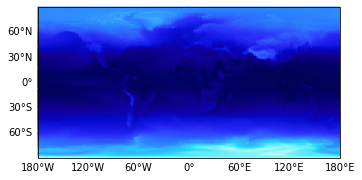# Gridded Datasets I#

GeoViews is designed to make full use of multidimensional gridded datasets stored in netCDF or other common formats, via the xarray and iris interfaces in HoloViews. This notebook will demonstrate how to load data using both of these data backends, along with some of their individual quirks. The data used in this notebook was originally shipped as part of the `SciTools/iris-sample-data` repository, but a smaller netCDF file is included as part of the GeoViews so that it can be used with xarray as well.

```import iris
import numpy as np
import xarray as xr
import holoviews as hv
import geoviews as gv
import geoviews.feature as gf

from cartopy import crs
from geoviews import opts

gv.extension('matplotlib')

gv.output(size=150)
```In this notebook we will primarily be working with xarray, but we will also load the same data using iris so that we can demonstrate that the two data backends are nearly equivalent.

### XArray#

As a first step we simply load the data using the `open_dataset` method xarray provides and have a look at the repr to get an overview what is in this dataset:

```xr_ensemble = xr.open_dataset('../data/ensemble.nc').load()
xr_ensemble
```
```<xarray.Dataset>
Dimensions:                  (time: 6, latitude: 145, longitude: 192, bnds: 2)
Coordinates:
* time                     (time) datetime64[ns] 2011-08-16T12:00:00 ... 20...
* latitude                 (latitude) float32 -90.0 -88.75 ... 88.75 90.0
* longitude                (longitude) float32 0.0 1.875 3.75 ... 356.2 358.1
forecast_period          (time) timedelta64[ns] 29 days 12:00:00 ... 182 ...
forecast_reference_time  datetime64[ns] 2011-07-18
Dimensions without coordinates: bnds
Data variables:
surface_temperature      (time, latitude, longitude) float32 214.0 ... 245.6
latitude_longitude       int32 -2147483647
time_bnds                (time, bnds) datetime64[ns] 2011-08-01 ... 2012-...
forecast_period_bnds     (time, bnds) float64 336.0 1.08e+03 ... 4.752e+03
Attributes:
source:       Data from Met Office Unified Model
um_version:   7.6
Conventions:  CF-1.5```

### Iris#

Similarly we can load the same dataset using Iris’ `load_cube` function and get a similar overview using the `summary` method.

```iris_ensemble = iris.load_cube('../data/ensemble.nc')
print(iris_ensemble.summary())
```
```surface_temperature / (K)           (time: 6; latitude: 145; longitude: 192)
Dimension coordinates:
time                             x            -               -
latitude                         -            x               -
longitude                        -            -               x
Auxiliary coordinates:
forecast_period                  x            -               -
Scalar coordinates:
forecast_reference_time     2011-07-18 00:00:00
Cell methods:
0                           time: mean (interval: 1 hour)
Attributes:
Conventions                 'CF-1.5'
STASH                       m01s00i024
source                      'Data from Met Office Unified Model'
um_version                  '7.6'
```

Describing the differences between these two libraries is well beyond the scope of this tutorial, but you can see from the summaries that the two libraries deal differently with both the bounds and with the actual data variables. Iris cubes support only a single data variable, while an xarray dataset can have any number of variables. In this case we are only interested in the `surface_temperature` dimension, indexed by `longitude`, `latitude` and `time`.

We can easily express this interest by wrapping the data in a GeoViews `Dataset` Element and declaring the key dimensions (`kdims`) and value dimensions (`vdims`). Note that the Iris interface is much smarter in the way it extracts the dimensions, so usually you will not have to supply them explicitly.

```kdims = ['time', 'longitude', 'latitude']
vdims = ['surface_temperature']

xr_dataset = gv.Dataset(xr_ensemble, kdims=kdims, vdims=vdims)
iris_dataset = gv.Dataset(iris_ensemble, kdims=kdims, vdims=vdims)
```

Now we can compare the repr of the two Elements:

```print(repr(xr_dataset))
print(repr(iris_dataset))
```
```:Dataset   [time,longitude,latitude]   (surface_temperature)
:Dataset   [time,longitude,latitude]   (surface_temperature)
```

Despite appearing identical, there are some internal differences, such as in the data types. xarray uses NumPy datetime64 types for dates, while iris will use simple floats:

```print("XArray time type: %s" % xr_dataset.get_dimension_type('time'))
print("Iris time type: %s" % iris_dataset.get_dimension_type('time'))
```
```XArray time type: <class 'numpy.datetime64'>
Iris time type: <class 'numpy.float64'>
```

To improve the formatting of dates on the xarray dataset we can set the formatter for datetime64 types:

```hv.Dimension.type_formatters[np.datetime64] = '%Y-%m-%d'
```

The other major differences in the way iris cubes are handled are in deducing various bits of metadata including the coordinate system, units, and formatters. Otherwise the two Dataset Elements will behave largely the same.

For either data backend, the `Dataset` object is not yet visualizable, because we have not chosen which dimensions to map onto which axes of a plot.

## A Simple example#

To visualize the datasets, in a single line of code we can specify that we want to view it as a collection of Images indexed by longitude and latitude (a HoloViews `HoloMap` of `gv.Image` elements):

```xr_dataset.to(gv.Image, ['longitude', 'latitude'])
```

You can see that the `time` dimension was automatically mapped to a slider, because we did not map it onto one of the other available dimensions (x, y, or color, in this case). You can drag the slider to view the surface temperature at different times. The example would work just the same for the `iris_dataset`.

Now let us load a cube showing the pre-industrial air temperature:

```pre_industrial = xr.open_dataset('../data/pre-industrial.nc').load()

air_temperature = gv.Dataset(pre_industrial, ['longitude', 'latitude'], 'air_temperature')
air_temperature
```
Dataset()
NameTypeValueBounds/ObjectsMode
cdims Dict OrderedDict()
datatype List ['dataframe', 'dictionary', 'grid', 'xar.. (0, None)
group String 'Dataset' constant
kdims List [Dimension('longitude'), Dimension('lati.. (0, None) constant
label String '' constant
name String 'Dataset03263' constant | nullable
vdims List [Dimension('air_temperature')] (0, None) constant

Note that we have the `air_temperature` available over `longitude` and `latitude` but not the `time` dimensions. As a result, this cube is a single frame (at right below) when visualized as an `Image`:

```gv.Image(air_temperature)
```The following more complicated example shows how complex interactive plots can be generated with relatively little code, and also demonstrates how different HoloViews elements can be combined together. In the following visualization, the black dot denotes a specific longitude, latitude location (0,10), and the curve is a sample of the `surface_temperature` at that location. The curve is unaffected by the `time` slider because it already lays out time along the x axis:

```temp_curve = hv.Curve(xr_dataset.select(longitude=0, latitude=10), kdims=['time'])
temp_map = xr_dataset.to(gv.Image,['longitude', 'latitude']) * gv.Points([(0,10)])
(temp_map + temp_curve).opts(
opts.Curve(aspect=2, xticks=4, xrotation=15),
opts.Points(color='k', global_extent=True))
```